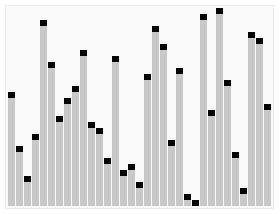# Integer - Multiplication (Product)

Integer multiplication

## Algo

### Computational problem

• Input: 2 n digit numbers x and y
• where n is large in the thousands or even more
• Output: the product x times y

### Assumption

• Primitive Operation (unit of performance): add or multiply 2 single digit numbers

### Application

• cryptographic application which has to manipulate very large numbers.

### Solution

$$\begin{array}{rrrrr} & & 1 & 2 & 3 \\ & & 4 & 5 & 6 \\ \hline & & 7 & 3 & 8 \\ & 6 & 1 & 5 & - \\ 4 & 9 & 2 & - & - \\ \hline 5 & 6 & 0 & 8 & 8 \end{array}$$ To form a partial products, we need

• $n$ operations for the multiplications. We multiplies 3 times each of the numbers 1, 2, and 3.
• $n$ operations (at most) for the carries. Carries are at most twice times the number of digits in the first number.

We need then at most $2n$ operations.

If we have $n$ partial products (The number of digits in the second number), the total is at most $2n^2$ operations to form all of them.

The final addition requires at most a comparable number of operations. $2n^2$ .

The number of operations for this Algorithm performs roughly $4n^2$ It's quadratic function in the input of length n.

#### Karatsuba Multiplication

Assumption: n is an even integer. If n is an odd integer, you can apply this exact same recursive approach to integer multiplication. If n was 9, the input numbers would be decomposed into a first five digits and a later four digits and you would proceed in exactly the same way.

$$\begin{array}{rrl} x & = & 10^{n/2} a + b \\ y & = & 10^{n/2} c + d \end{array}$$ where:

• a,b,c,d are $n/2$ digit numbers. example: a=56, b=78, c=12, d=34

Then

$$\begin{array}{rrl} x.y & = &(10^{n/2} a + b)(10^{n/2} c + d) \\ & = & 10^{n} ac + 10^{n/2}(ad+bc) + bd \end{array}$$ Idea :

• recursively compute ac, ad, bc, bd,
1. Recursively compute ac
2. Recursively compute bd
3. Recursively compute (a+b)(c+d) = ac + bd + ad + bc.
4. Then Gauss’Trick: (3)–(1)–(2)= ad + bc

Recursivity base case: If the input (n) is sufficiently small (one digit), then compute the answer rather than recursing further.

• then compute $x.y$

Recommended PagesAlgorithm

An is a (procedure|method) for solving a problem. If there exists an algorithm, the function that performs it is called computable. Study of algorithms dates at least to Euclid and were formalized by...